# Various ways of writing Nesterov's acceleration

Nestorov’s acceleration is usually presented in the following form (AGM1)where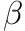is the gradient Lipschitz parameter of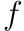.

The update is known to be notoriously opaque. There are alternatives ways of rewriting the first-order scheme, however, two of which we will cover here.

### 1.1 Acceleration from primal dual perspective

We want to rewrite AGM1 as the following scheme (called AGM2)whereis-smooth,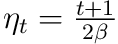and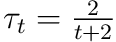. This is a linear coupling of a small step size update (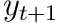) and a large step size update (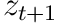).

##### Derivation

Notice that the definition foris already the same which leaves us with verifying the update for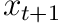and. Let’s start by assuming that the correct update for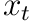exists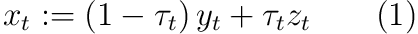This leaves us with checking whether this leads to the right definition of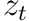. That is, we need to show that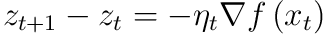according to the AGM2 update. First we make a guess about the relationship between parameters,Using this after isolatingin eq. 1 gives us,Expanding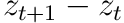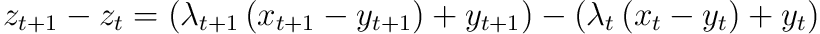Using definition ofin AGM1,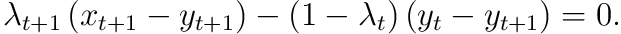We can cancel terms by subtracting it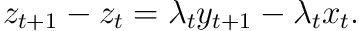We can further develop by using the update for,What is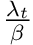? If it is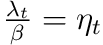then we are done.So we are weirdly enough off by one since it should be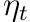Source: potential function paper, p. 468.

### 1.2 As momentum

NAG can be written as a momentum scheme where the gradient is queried at “a future point”.##### DerivationNow, naturally define the momentum as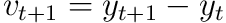and the parameter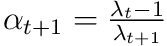for convenience. This lets us write the above as,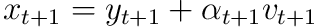This definition can be used in the update forFinally using this form ofin the definition for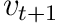we obtain the desired momentum updateSimply writingusing the definition ofcompletes the schemeSource: acceleration as momentum (appendix).